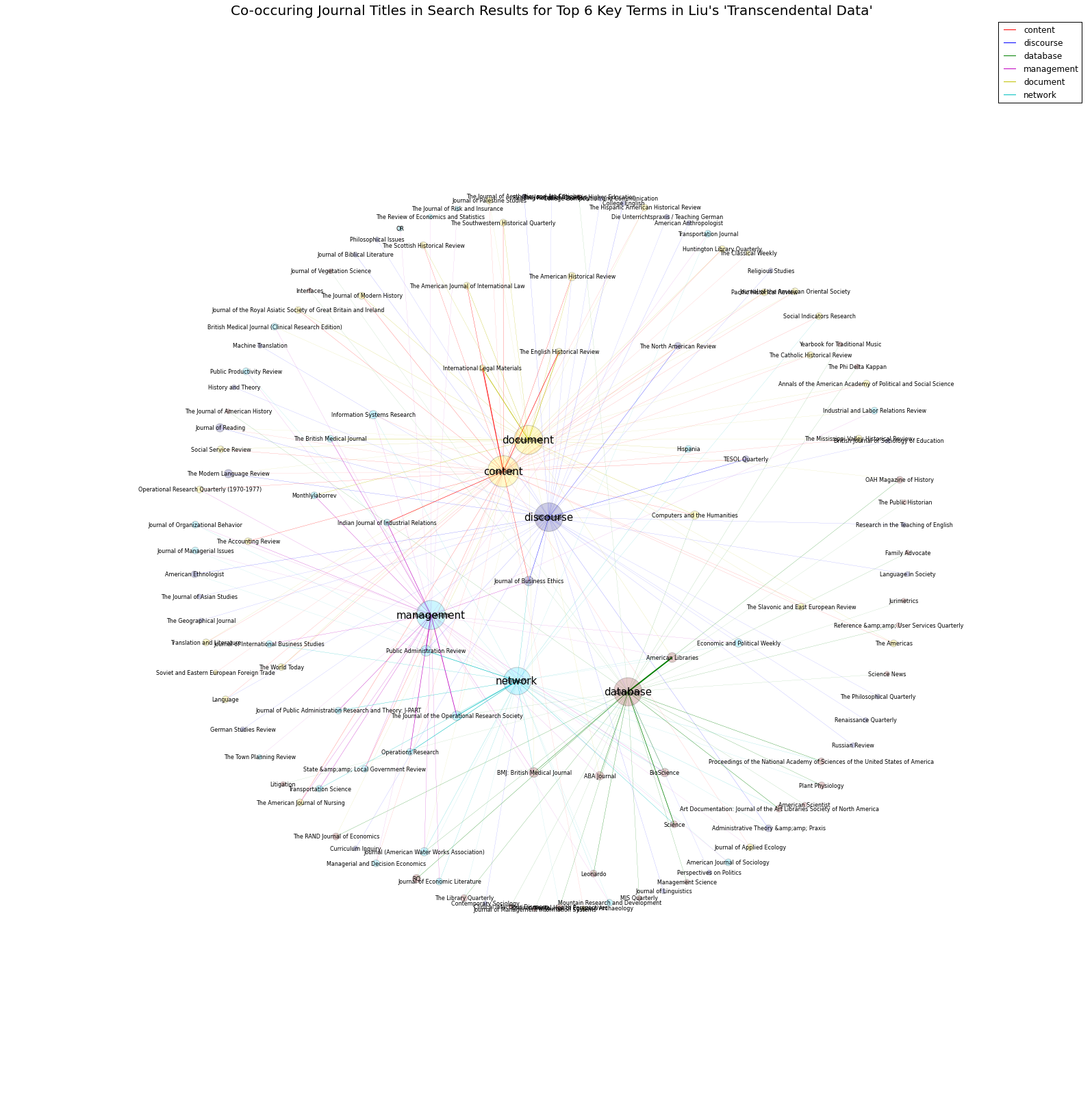# Mining your Search Results with JSTOR Data for Research:¶

Mapping the shape of digital interdisciplinarity

In this tutorial, we will:

• Import Search metadata from JSTOR Data for Research
• Create a network of search terms and academic journals that appear in the searches.
• Visualize the network graph of the search results in order to view something like the shape of interdisciplinarity in Liu's essay.

### Import necessary libraries and functions¶

In :
import re
import os.path
from os import listdir
from glob import glob
import codecs
from collections import defaultdict
import math
import community
##functions for reading file and filename
def remove_ext(filename):
file, ext = os.path.splitext(filename)
return file
def remove_dir(filepath):
direct = os.path.basename(filepath)
return direct
def get_filename(filepath):
file = remove_ext(filepath)
file = remove_dir(file)
return file
"""Returns the full raw text of the corpus document, in a single string"""
f = open(filepath)
f = codecs.open(filepath, "r", "utf-8") #open(filepath)
f.close()
return text
def find_corpus_files(corpusdirectory):
"""Returns a list of all filenames of corpus files"""
return glob(corpusdirectory + "/*.csv")
def texts_containing(word, doc_list):
'''Takes a word and a list of documents (stored as word lists).
Returns number of words in a list of documents.'''
count = 0
for doc in doc_list:
if word in doc:
count += 1
return count
def idf(word, doc_list):
'''Takes a word and a lists of documents, and returns the inverse of the number of
documents that the word appears in.'''
return math.log(len(doc_list)/ (1 + texts_containing(word, doc_list)))
## importing file chunk
corpusdirectory = "liu_transcendental_data"


### Import JSTOR CSVs¶

We are retrieving the the search results 15 bigram searches using the top 6 key terms for Liu's 2004 Critical Inquiry article, "Transcendental Data: Toward a Cultureal History and Aesthetics of the New Encoded Discourse," (Autumn, 2004). JSTOR's top 6 key terms are: database, discourse, content, management, document, and network.

In :
journal_edgelist = []
journal_dict = defaultdict(list)
for i in find_corpus_files(corpusdirectory):
query = get_filename(i).split('_')
for line in text.split("\n"):
if not line.strip():
continue
if "Journal,ARTICLE_COUNT" in line:
continue
else:
x = line.encode('utf8').decode('utf8')
journal,number = x.split(",", 1) ##, 1)
for i in query:
journal_dict[i].append((journal, number))


#### Check that we have the journals for each search term.¶

In :
journal_dict.keys()

Out:
dict_keys(['management', 'database', 'discourse', 'network', 'content', 'document'])

### Weight the search results using the Term Frequency-Inverse Document Frequency Measure¶

This measure is a popular and conventional measure from Information Retrieval (the field responsible for many search algorithms) used to score terms in a document based on their relative frequency in that document and their inverse frequency in the larger corpus. It thus give higher scores to terms (or here journals) that often appear in one document (or search) and not in others, so as to reduce the noise from terms/journals that are frequent across all documents/searches.

In :
journals = []
journal_corpus = []
for query, value in journal_dict.items():
query_list = []
for journal, num in value:
query_list.append(journal)
journals.append(journal)
journal_corpus.append(query_list)
journals = list(set(journals))

In :
journal_idf = defaultdict(int)
for journal in journals:
journal_idf[journal] = idf(journal, journal_corpus) + 1


#### Check the Inverse Document Frequency of one Journal¶

In :
journal_idf['The American Historical Review']

Out:
0.8458493201727416

#### First we we will get the TF-IDF score for each journal¶

In :
journal_network2 = []
query_list = []
for query, value in journal_dict.items():
total_value = 0
#for key, value in query.items():
query_list.append(query)
for journal, num in value:
total_value += int(num)
for journal, num in value:
tf_idf_score = (int(num)/total_value) *journal_idf[journal]
journal_network2.append((query, journal, tf_idf_score))


#### Now we will get the average score & re-produce the network with a filter to take only journals that have scores 2.5 times greater than the average score¶

In :
total_score = 0
for item in journal_network2:
total_score += item
avg = total_score/len(journal_network2)

In :
journal_network2 = []
query_list = []
for query, value in journal_dict.items():
total_value = 0
#for key, value in query.items():
query_list.append(query)
for journal, num in value:
total_value += int(num)
for journal, num in value:
tf_idf_score = (int(num)/total_value) *journal_idf[journal]
if tf_idf_score > avg*2.5:
journal_network2.append((query, journal, tf_idf_score))


### Create network graph¶

In :
import networkx as nx
import matplotlib.pyplot as plt
import matplotlib
%matplotlib inline

G = nx.Graph()
for i in journal_network2:
wt = i*20
pos = nx.spring_layout(G, scale=4)


### Alter size of network nodes according to number of edges¶

In :
nodes = []
degrees = []
for node in G.nodes():
node_degree = 0
for edge in G.edges():
if node in edge:
node_degree += 1
node_degree = node_degree*50
degrees.append(node_degree)
nodes.append(node)


#### Modify edge colors in network¶

In :
colors = []
query_edges = []
for edge in G.edges():
if 'content' in edge:
colors.append('r')
query_edges.append(edge)
elif 'discourse' in edge:
colors.append('b')
query_edges.append(edge)
elif 'database' in edge:
colors.append('g')
query_edges.append(edge)
elif 'management' in edge:
colors.append('m')
query_edges.append(edge)
elif 'document' in edge:
colors.append('y')
query_edges.append(edge)
elif 'network' in edge:
colors.append('c')
query_edges.append(edge)
color_labels = ['r', 'b', 'g', 'm', 'y', 'c']
edge_labels = ['content', 'discourse', 'database', 'management', 'document', 'network']


## Plot & Save the graph as png file¶

In :
plt.figure(figsize=(28,28), dpi=100)
g = G.subgraph([label for label, _ in pos.items() if label in query_list]) # if "topic " in topic])
nx.draw_networkx_labels(g, pos,  font_color='black', font_size=15, )
g = G.subgraph([journal for journal, value in pos.items() if journal not in query_list and value > avg*2 or
value>avg*2])
nx.draw_networkx_labels(g, pos, label_pos=1, font_size=8)

## Algorithm for automatically detecting communities in the network
part = community.best_partition(G)
values = [part.get(node) for node in G.nodes()]
nx.draw_networkx_nodes(G, pos, node_list=nodes, cmap = plt.get_cmap('jet'), node_color = values, node_size=degrees,
with_labels=False, alpha=0.2)

edges = G.edges()
weights = [G[u][v]['weight'] for u,v in edges]

# plot edges
nx.draw_networkx_edges(G, pos, edgelist=G.edges(), alpha=1, edge_color=colors, width=weights)

f = plt.figure(1)
for color, edge in zip(color_labels, edge_labels):
ax.plot(,,color=color,label=edge)

plt.axis('off')
plt.title("Co-occuring Journal Titles in Search Results for Top 6 Key Terms in Liu's 'Transcendental Data'",
fontsize=20)
plt.legend()
plt.savefig('search_network.png', dpi=150, orientation='landscape', bbox_inches='tight')
plt.show()

/Users/collin/anaconda/lib/python3.4/site-packages/matplotlib/collections.py:650: FutureWarning: elementwise comparison failed; returning scalar instead, but in the future will perform elementwise comparison
if self._edgecolors_original != str('face'):
/Users/collin/anaconda/lib/python3.4/site-packages/matplotlib/collections.py:590: FutureWarning: elementwise comparison failed; returning scalar instead, but in the future will perform elementwise comparison
if self._edgecolors == str('face'):#### Check out some basic information on our graph¶

In [ ]:
print(nx.info(G))

In [ ]:
print(community.modularity(part,G))